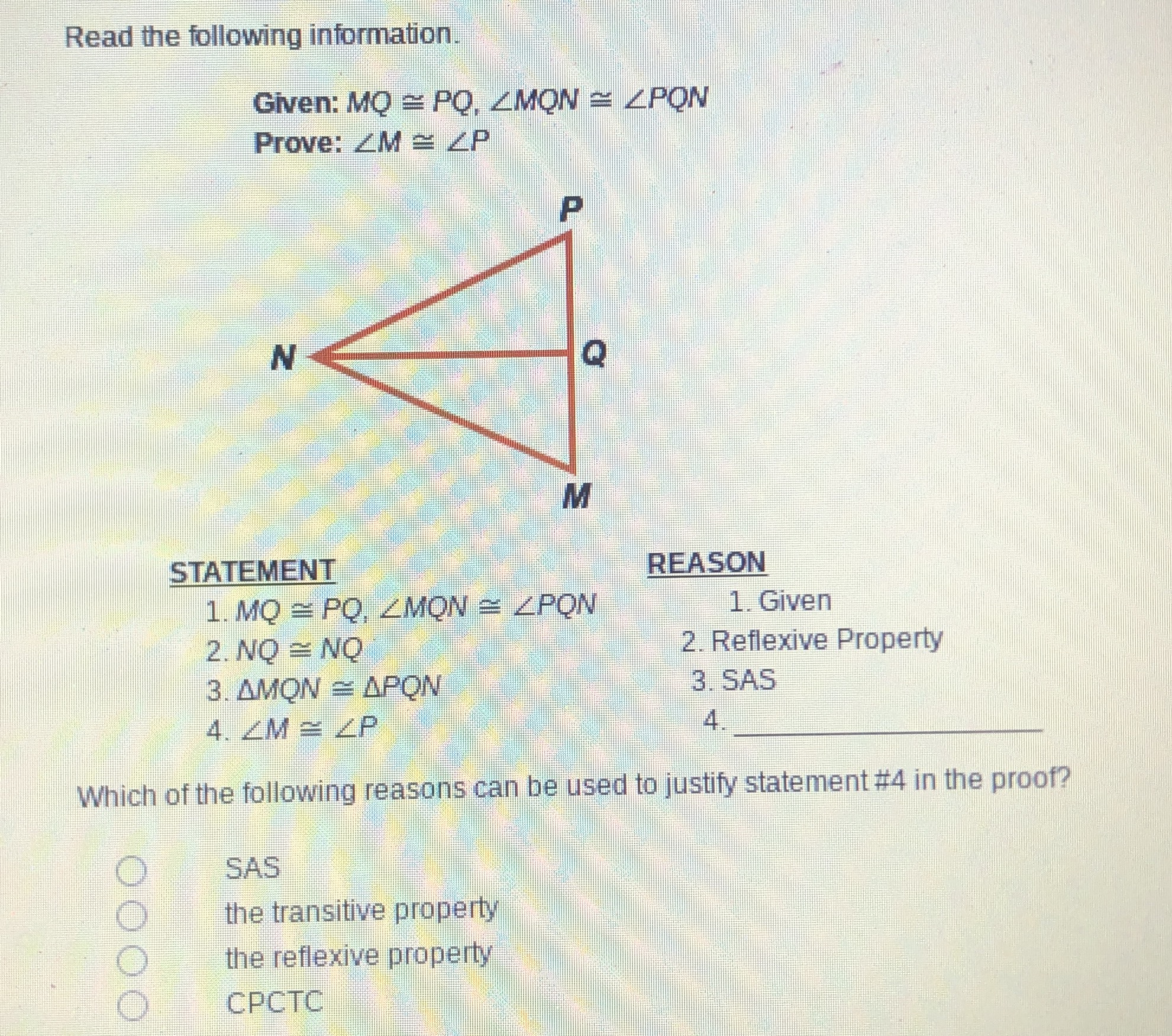### Still have math questions?

Trigonometry
QuestionRead the following information. Given: $$M Q \cong P Q , \angle M Q N \cong \angle P Q N$$

Prove: $$\angle M \cong \angle P$$ STATEMENT 1.MO $$\cong P Q , \angle M O N \cong \angle P Q N$$

2. NO $$\cong N O$$ 3. $$\triangle M O N \cong \triangle P O N$$

$$4 . \angle M \cong \angle P$$ REASON 1. Given 2. Reflexive Property 3. SAS 4.